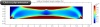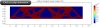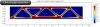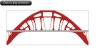借助拓扑优化找出结构的最优设计

2015年 9月 23日

简支梁示例通过正确的优化方法找出结构的最优设计

• 如果 \alpha 是几何的一组控制参数（如长度或高度），这就是一个参数化优化问题。
• 如果 \alpha 控制了几何的外部曲线，这将是形状优化问题。
• 如果 \alpha 是确定几何内某点为空心或实心的函数，则是拓扑优化问题。

E(X)= \rho_{design} (X) E_0

\displaystyle Ws_{total}=\int_\Omega Ws \ d\Omega=\int_\Omega \frac{1}{2} \sigma: \varepsilon\ d\Omega

\min_{\rho_{design}}⁡\int_\Omega \frac{1}{2} \sigma (\rho_{design}): \varepsilon\ d\Omega

在 COMSOL Multiphysics 中进行拓扑优化\int_\Omega \rho \leq 0.5M_0 \Leftrightarrow \int_\Omega \rho_{design} \leq 0.5V_0E(X)=(\rho_{design})^p E_0\int_\Omega |\nabla \rho_{design}|^2 \ d\Omega

\min_{\rho_{design}} \ \ {⁡q\int_\Omega \frac{Ws}{Ws0} \ d\Omega + (1-q)\frac{h_0 h_{max}}{A}\int_\Omega |\nabla \rho_{design}|^2 \ d\Omega}
s.t. ~ \int_\Omega ρ_{design} \leq 0.5V_0在水面上设计一座桥参考文献

1. “Optimal shape design as a material distribution problem”, by M.P. Bendsøe.
2. Topology Optimization: Theory, Methods, and Applications, by M.P. Bendsøe and O. Sigmund.

评论 (5)

留言杰 王
2018-06-19Tengyue Gao
2018-10-12paul wood
2018-11-08

In the Model Builder window, under Component 1 (comp1)right-click Definitions and
choose Topology Optimization>Density Model.珣 刘
2019-01-252019-05-28

lxy00428@163.com